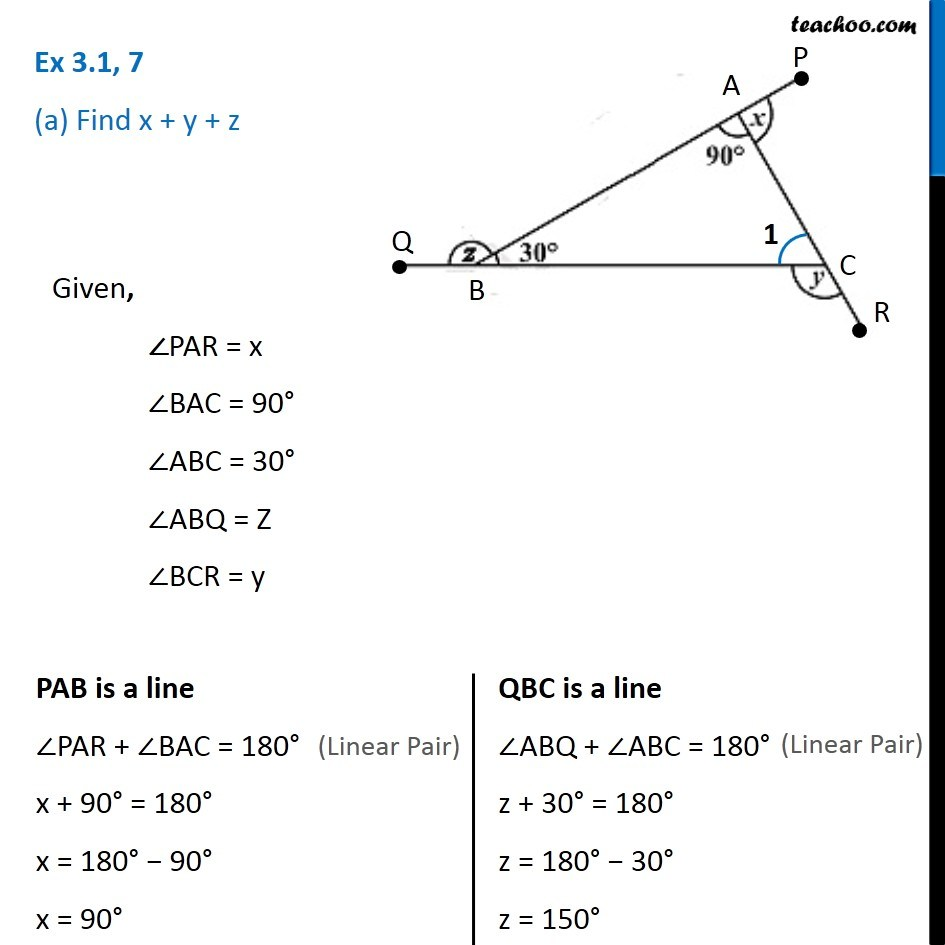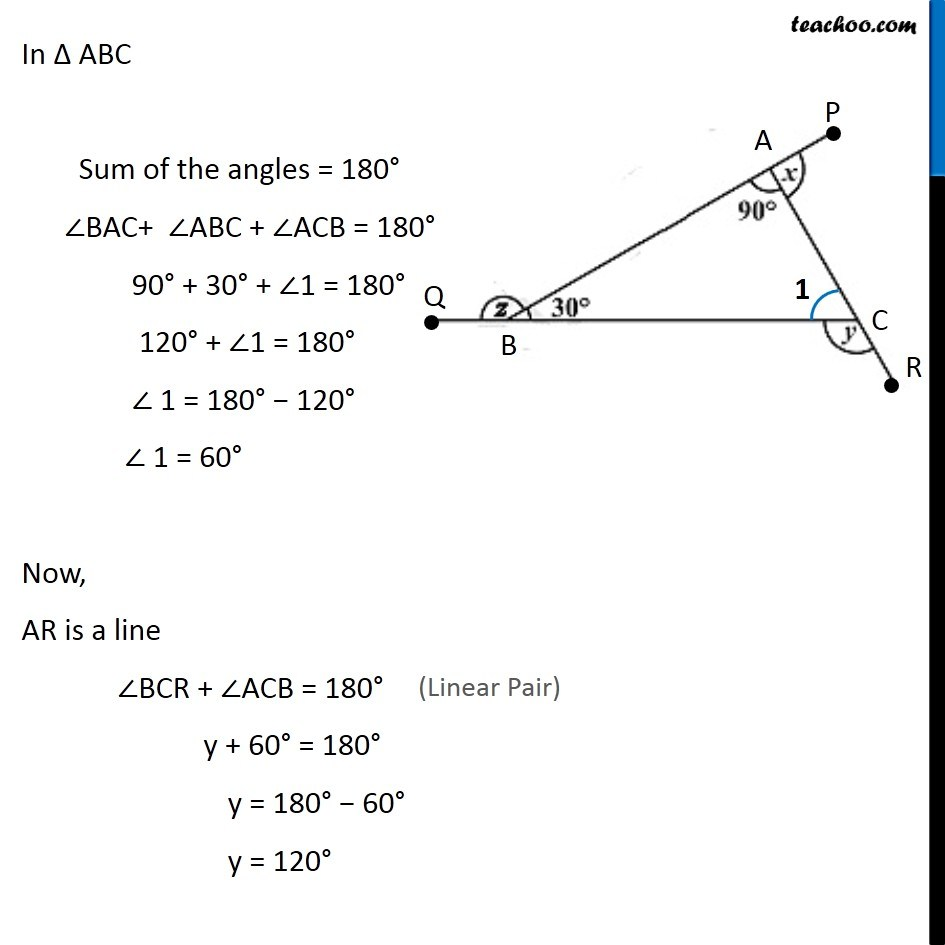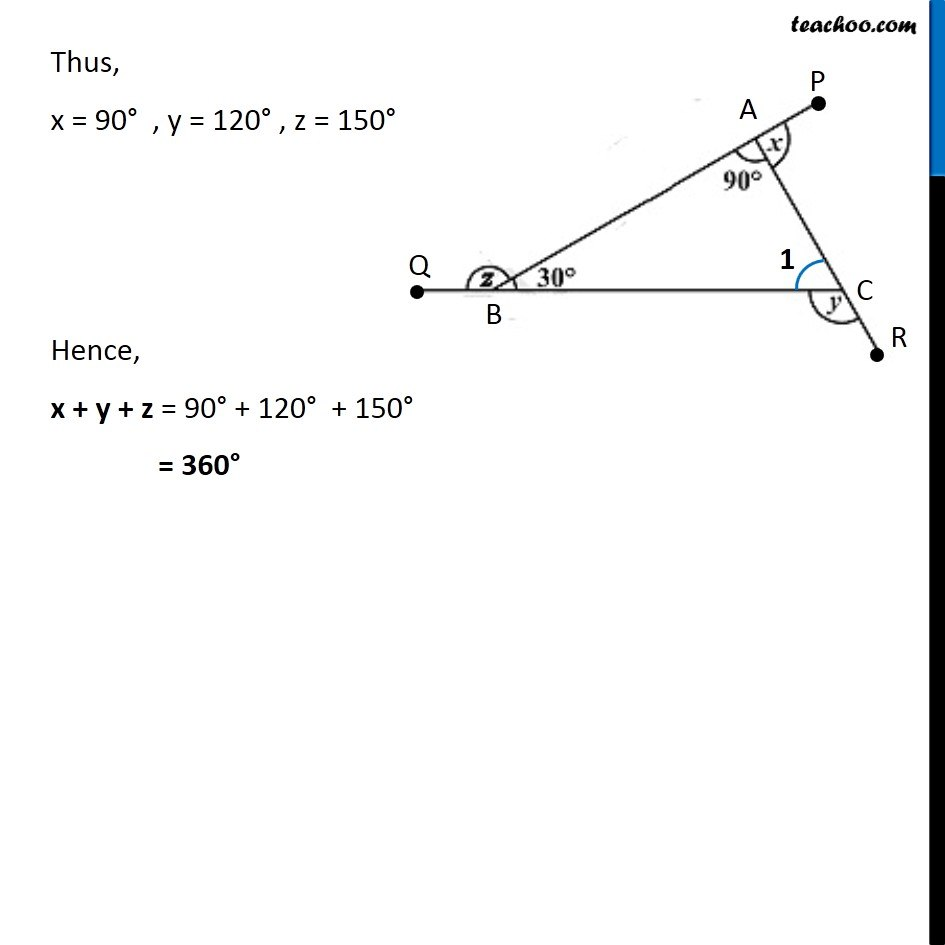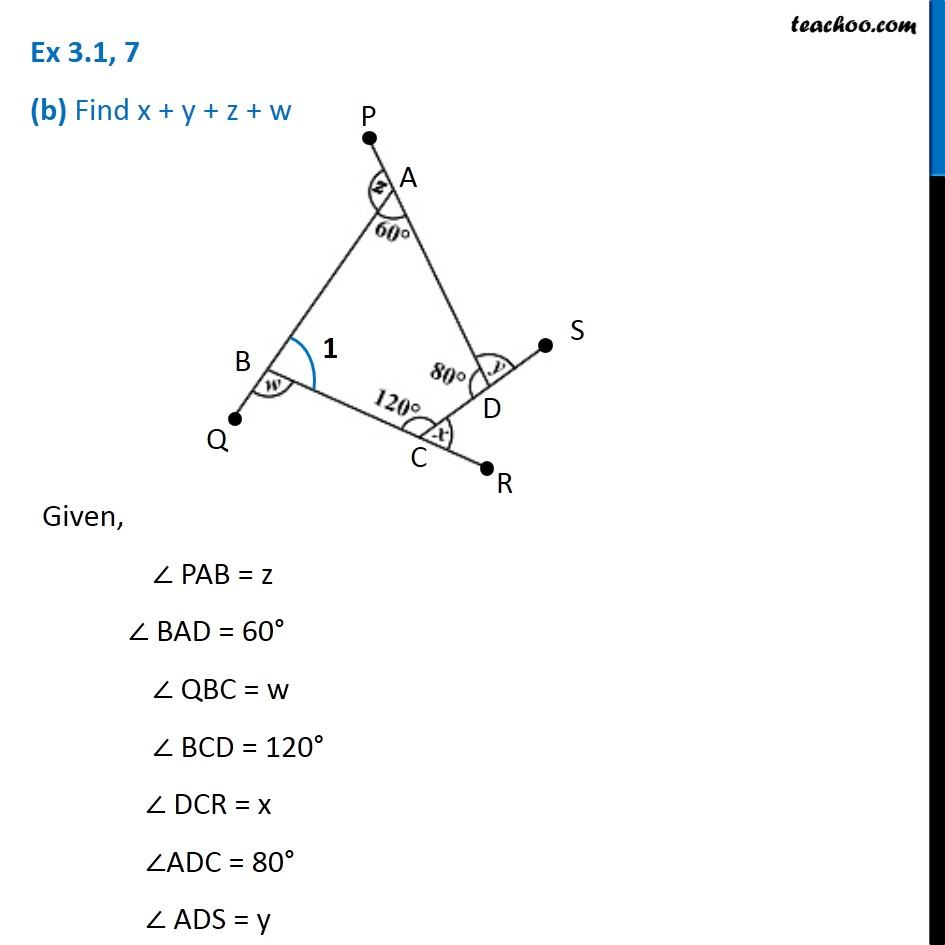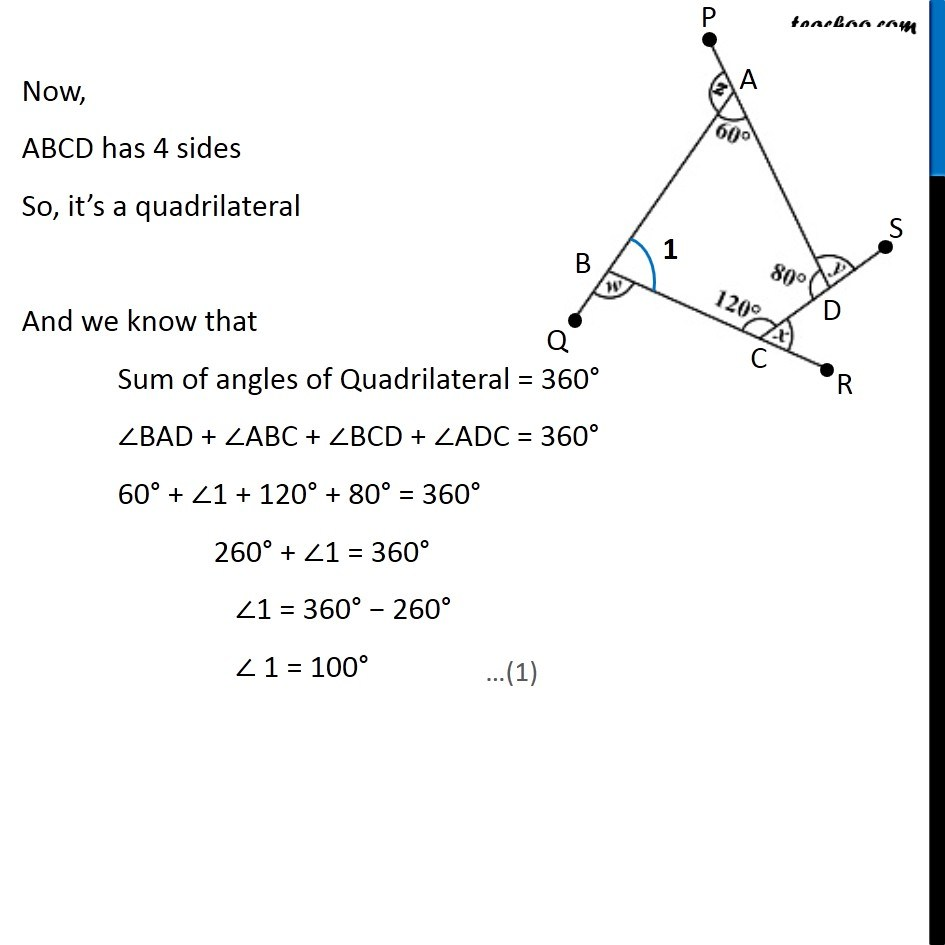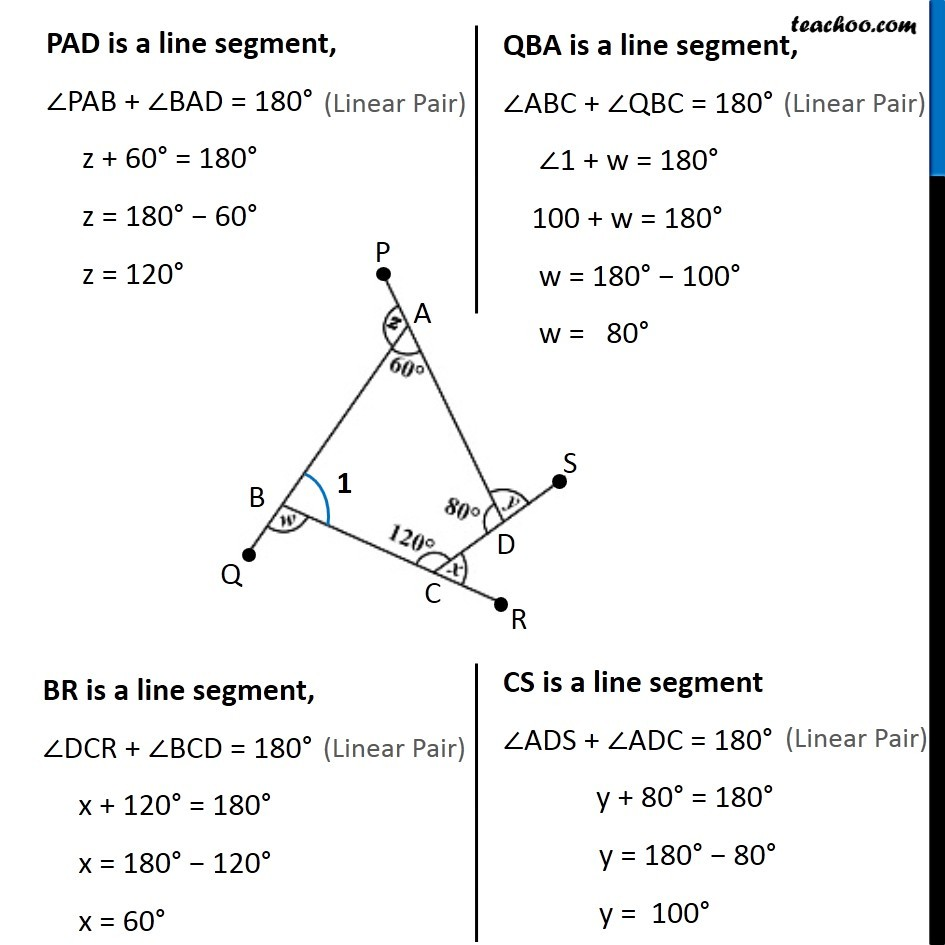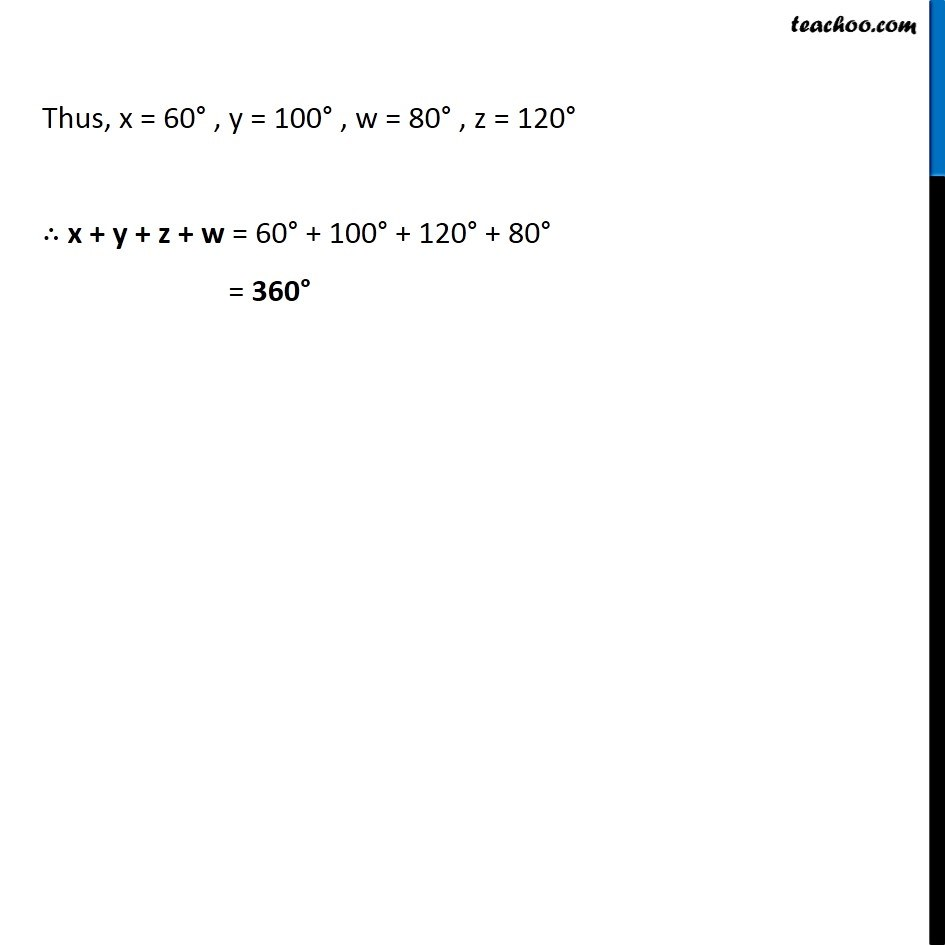1. Chapter 3 Class 8 Understanding Quadrilaterals
2. Concept wise
3. Polygons

Transcript

Ex 3.1, 7 (a) Find x + y + zGiven, ∠PAR = x ∠BAC = 90° ∠ABC = 30° ∠ABQ = Z ∠BCR = y PAB is a line ∠PAR + ∠BAC = 180° x + 90° = 180° x = 180° − 90° x = 90° QBC is a line ∠ABQ + ∠ABC = 180° z + 30° = 180° z = 180° − 30° z = 150° In ∆ ABC Sum of the angles = 180° ∠BAC+ ∠ABC + ∠ACB = 180° 90° + 30° + ∠1 = 180° 120° + ∠1 = 180° ∠ 1 = 180° − 120° ∠ 1 = 60° Now, AR is a line ∠BCR + ∠ACB = 180° y + 60° = 180° y = 180° − 60° y = 120° Thus, x = 90° , y = 120° , z = 150° Hence, x + y + z = 90° + 120° + 150° = 360° Ex 3.1, 7 (b) Find x + y + z + w Given, ∠ PAB = z ∠ BAD = 60° ∠ QBC = w ∠ BCD = 120° ∠ DCR = x ∠ADC = 80° ∠ ADS = y Now, ABCD has 4 sides So, it’s a quadrilateral And we know that Sum of angles of Quadrilateral = 360° ∠BAD + ∠ABC + ∠BCD + ∠ADC = 360° 60° + ∠1 + 120° + 80° = 360° 260° + ∠1 = 360° ∠1 = 360° − 260° ∠ 1 = 100° PAD is a line segment, ∠PAB + ∠BAD = 180° z + 60° = 180° z = 180° − 60° z = 120° QBA is a line segment, ∠ABC + ∠QBC = 180° ∠1 + w = 180° 100 + w = 180° w = 180° − 100° w = 80° BR is a line segment, ∠DCR + ∠BCD = 180° x + 120° = 180° x = 180° − 120° x = 60° CS is a line segment ∠ADS + ∠ADC = 180° y + 80° = 180° y = 180° − 80° y = 100° Thus, x = 60° , y = 100° , w = 80° , z = 120° ∴ x + y + z + w = 60° + 100° + 120° + 80° = 360°

Polygons

About the AuthorDavneet Singh
Davneet Singh is a graduate from Indian Institute of Technology, Kanpur. He has been teaching from the past 9 years. He provides courses for Maths and Science at Teachoo.